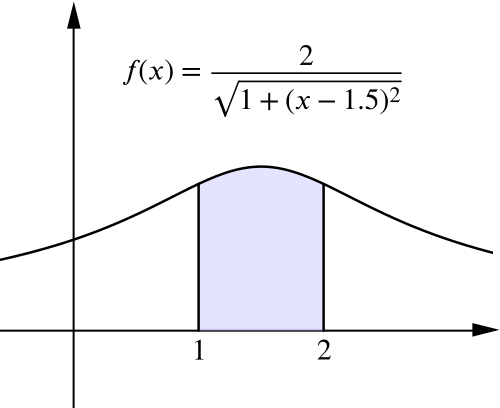### Introducing Calculus

Food for thought

What can you say about the area under each function on the two cards below, between $x=1$ and $x=2$?
 (a) $f(x)=\left(4-x^2\right)^{\frac{1}{2}}$ (b)